Ask your WordPress questions! Pay money and get answers fast!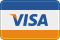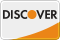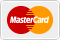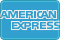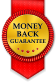# insert custom data from non wordpress database into wp posts WordPress

• SOLVED

I have a non wordpress database that contains products, I need to import these products into wordpress posts and create a custom field containing the products unique reference code. I have the following code that nearly works but my custom field just contains the same information for every entry. I'm a bit of noob with php and this is really bugging me as to how to do this.

The problem is I require 3 custom fields for each product, where the product title and the product id and description are populated from my non wordpress database and these create a wordpress post. The non wordpress database contains 1200 products and really need this wordpress post population to be fully automatic because as I will be getting a update of the non wordpress database on a weekly basis and just want to find a simple solution.

``` \$results=mysql_query("SELECT * FROM nonwordpressdatabase WHERE AB_USERID =12345") or die('Query Failed: '.mysql_error()); echo "Executed Query by kennyboy7<br/>\n"; \$i = 0; while (\$row = mysql_fetch_array(\$results,MYSQL_ASSOC)) { \$post = array(); \$post['post_status'] = 'publish'; \$post['post_category'] = array(1); \$post['post_date'] = date('Y-m-d H:i:s',strtotime(\$row['newsitem_date'])); \$post['post_title'] = \$row['AB_NAME']; \$post['post_content'] = \$row['AB_DESCR']; // this is where the problem occurs and it's not looping the same as the posts \$product_code = \$row['AB_INDEX']; \$product_name = \$row['AB_NAME']; \$posts[\$i] = \$post; \$i++; } mysql_free_result(\$results); mysql_close(\$conn); require('./wp-load.php'); foreach (\$posts as \$post) { wp_insert_post(\$post); \$post_id = wp_insert_post(\$post); add_post_meta(\$post_id, 'product_code',\$product_code, true); add_post_meta(\$post_id, 'product_name',\$product_name, true); } ```

The above code is a created from various articles I've found on the web and if it is incorrect or can be re-written to solve this problem then please feel free to do so.

2011-08-25

``` \$results=mysql_query("SELECT * FROM nonwordpressdatabase WHERE AB_USERID =12345") or die('Query Failed: '.mysql_error()); echo "Executed Query by kennyboy7<br/>\n"; \$i = 0; while (\$row = mysql_fetch_array(\$results,MYSQL_ASSOC)) { \$post = array(); \$post['post_status'] = 'publish'; \$post['post_category'] = array(1); \$post['post_date'] = date('Y-m-d H:i:s',strtotime(\$row['newsitem_date'])); \$post['post_title'] = \$row['AB_NAME']; \$post['post_content'] = \$row['AB_DESCR']; // this is where the problem occurs and it's not looping the same as the posts \$post['product_code'] = \$row['AB_INDEX']; \$post['product_name'] = \$row['AB_NAME']; \$posts[\$i] = \$post; \$i++; } mysql_free_result(\$results); mysql_close(\$conn); require('./wp-load.php'); foreach (\$posts as \$post) { wp_insert_post(\$post); \$post_id = wp_insert_post(\$post); add_post_meta(\$post_id, 'product_code',\$post['product_code'], true); add_post_meta(\$post_id, 'product_name',\$post['product_name'], true); }```

2011-08-25

This should work:

<?php
``` \$results=mysql_query("SELECT * FROM nonwordpressdatabase WHERE AB_USERID =12345") or die('Query Failed: '.mysql_error()); echo "Executed Query by kennyboy7<br/>\n"; \$i = 0; while (\$row = mysql_fetch_array(\$results,MYSQL_ASSOC)) { \$post = array(); \$post['post_status'] = 'publish'; \$post['post_category'] = array(1); \$post['post_date'] = date('Y-m-d H:i:s',strtotime(\$row['newsitem_date'])); \$post['post_title'] = \$row['AB_NAME']; \$post['post_content'] = \$row['AB_DESCR']; \$post['product_code'] = \$row['AB_INDEX']; \$post['product_name'] = \$row['AB_NAME']; \$posts[\$i] = \$post; \$i++; } mysql_free_result(\$results); mysql_close(\$conn); require('./wp-load.php'); foreach (\$posts as \$post) { \$product_code = \$post['product_code']; \$product_name = \$post['poduct_name']; unset(\$post['product_code']); unset(\$post['product_name']); \$post_id = wp_insert_post(\$post); add_post_meta(\$post_id, 'product_code',\$product_code, true); add_post_meta(\$post_id, 'product_name',\$product_name, true); } ```

2011-08-25

Hello

First you have 2 times "wp_insert_post()", i don't think this is usefull.
Then you create an array with posts and then use a foreach after that...mmm

i coded this <em>(do more with less code)</em> :

```\$results = mysql_query( "SELECT * FROM nonwordpressdatabase WHERE AB_USERID =12345" ) or die( 'Query Failed: ' . mysql_error() ); echo "Executed Query by kennyboy7<br/>\n"; require( './wp-load.php' ); while ( \$row = mysql_fetch_array( \$results, MYSQL_ASSOC ) ) { \$post = array(); \$post['post_status'] = 'publish'; \$post['post_category'] = array(1); \$post['post_date'] = date( 'Y-m-d H:i:s', strtotime( \$row['newsitem_date'] ) ); \$post['post_title'] = \$row['AB_NAME']; \$post['post_content'] = \$row['AB_DESCR']; \$post_id = wp_insert_post( \$post ); add_post_meta( \$post_id, 'product_code', \$row['AB_INDEX'], true ); add_post_meta( \$post_id, 'product_name', \$row['AB_NAME'], true ); } mysql_free_result( \$results ); mysql_close( \$conn ); ```

See you soon !

``` \$results=mysql_query("SELECT * FROM nonwordpressdatabase WHERE AB_USERID =12345") or die('Query Failed: '.mysql_error()); echo "Executed Query by kennyboy7<br/>\n"; \$i = 0; while (\$row = mysql_fetch_array(\$results,MYSQL_ASSOC)) { \$post = array(); \$post['post_status'] = 'publish'; \$post['post_category'] = array(1); \$post['post_date'] = date('Y-m-d H:i:s',strtotime(\$row['newsitem_date'])); \$post['post_title'] = \$row['AB_NAME']; \$post['post_content'] = \$row['AB_DESCR']; // this is where the problem occurs and it's not looping the same as the posts \$post['product_code'] = \$row['AB_INDEX']; \$post['product_name'] = \$row['AB_NAME']; \$posts[\$i] = \$post; \$i++; } mysql_free_result(\$results); mysql_close(\$conn); require('./wp-load.php'); foreach (\$posts as \$post) { wp_insert_post(\$post); \$post_id = wp_insert_post(\$post); add_post_meta(\$post_id, 'product_code',\$post['product_code'], true); add_post_meta(\$post_id, 'product_name',\$post['product_name'], true); } ```